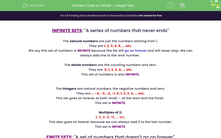# Recognise Infinite and Finite Sets of Numbers

In this activity, students will investigate the infinite nature of various sets of integers and the finite nature of other subgroups.Key stage:  KS 3

Curriculum topic:   Number

Curriculum subtopic:   Appreciate Infinite Sets

Popular topics:   Factors worksheets

Difficulty level:#### Worksheet Overview

An infinite set is a series of numbers that never ends.

The natural numbers are just the numbers starting from 1.

They are 1, 2, 3, 4, 5, ... etc.

We say this set of numbers is infinite because the list will go on forever and will never end. We can always add one to the 'end' number.

The whole numbers are the counting numbers and zero.

They are  0, 1, 2, 3, 4, ... etc.

This set of numbers is also infinite.

The integers are natural numbers, the negative numbers and zero.

They are ... -4, -3, -2, -1, 0, 1, 2, 3, 4, ... etc.

This set goes on forever at both 'ends' – at the start and the finish.

This set is infinite.

Multiples of 3:

3, 6, 9, 12, 15, ... etc.

This also goes on forever because we can always add 3 to the last number.

This set is infinite.A finite set is a set of numbers that doesn't go on forever.

The factors of 12:

They are 1, 2, 3, 4, 6 and 12.

There are no more.

This set is finite as it does not go on forever. It has an end.

Look at the multiples of 12 less than 50.

They are 12, 24, 36, and 48.

There are no more, because we cannot go past 50.

This set is finite as it does not go on forever.What a lot of different types of number to remember!

If you need  a reminder while doing the questions, just click on the red button on the screen and it will show you this introduction again.

Let's get started on some questions.

### What is EdPlace?

We're your National Curriculum aligned online education content provider helping each child succeed in English, maths and science from year 1 to GCSE. With an EdPlace account you’ll be able to track and measure progress, helping each child achieve their best. We build confidence and attainment by personalising each child’s learning at a level that suits them.

Get started